# Snub dodecahedron

﻿
Snub dodecahedron
Snub dodecahedron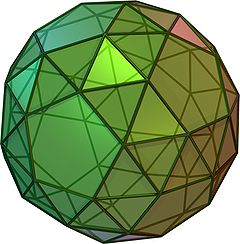Type Archimedean solid
Uniform polyhedron
Elements F = 92, E = 150, V = 60 (χ = 2)
Faces by sides (20+60){3}+12{5}
Schläfli symbol s{5,3}
Wythoff symbol | 2 3 5
Coxeter-DynkinSymmetry I, [5,3]+, (532)
Dihedral Angle
References U29, C32, W18
Properties Semiregular convex chiral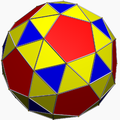Colored faces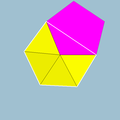3.3.3.3.5
(Vertex figure)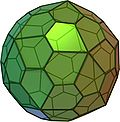Pentagonal hexecontahedron
(dual polyhedron)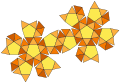Net

In geometry, the snub dodecahedron, or snub icosidodecahedron, is an Archimedean solid, one of thirteen convex isogonal nonprismatic solids constructed by two or more types of regular polygon faces.

The snub dodecahedron has 92 faces (the most of any convex uniform polyhedron other than prisms and antiprisms), of which 12 are pentagons and the other 80 are equilateral triangles. It also has 150 edges, and 60 vertices. It has two distinct forms, which are mirror images (or "enantiomorphs") of each other.

## Geometric relations

The snub dodecahedron can be generated by taking the twelve pentagonal faces of the dodecahedron and pulling them outward so they no longer touch. At a proper distance this can create the rhombicosidodecahedron by filling in square faces between the divided edges and triangle faces between the divided vertices. But for the snub form, only add the triangle faces and leave the square gaps empty. Then apply an equal rotation to the centers of the pentagons and triangles, continuing the rotation until the gaps can be filled by two equilateral triangles.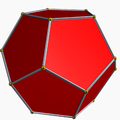Dodecahedron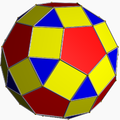Rhombicosidodecahedron (Expanded dodecahedron)

The snub dodecahedron can also be derived from the truncated icosidodecahedron by the process of alternation. Sixty of the vertices of the truncated icosidodecahedron form a polyhedron topologically equivalent to one snub dodecahedron; the remaining 60 form its mirror-image. The resulting polyhedron is vertex-transitive but not uniform, because its edges are of unequal lengths; some deformation is required to transform it into a uniform polyhedron.

Archimedes, an ancient Greek who showed major interest in polyhedral shapes, wrote a treatise on thirteen semi-regular solids. The snub dodecahedron is one of them.

## Cartesian coordinates

Cartesian coordinates for the vertices of a snub dodecahedron are all the even permutations of

(±2α, ±2, ±2β),
(±(α+β/τ+τ), ±(-ατ+β+1/τ), ±(α/τ+βτ-1)),
(±(-α/τ+βτ+1), ±(-α+β/τ-τ), ±(ατ+β-1/τ)),
(±(-α/τ+βτ-1), ±(α-β/τ-τ), ±(ατ+β+1/τ)) and
(±(α+β/τ-τ), ±(ατ-β+1/τ), ±(α/τ+βτ+1)),

with an even number of plus signs, where

α = ξ-1/ξ

and

β = ξτ+τ2+τ/ξ,

where τ = (1+√5)/2 is the golden ratio and ξ is the real solution to ξ3-2ξ=τ, which is the number:$\xi = \sqrt{\frac{\tau}{2} + \frac{1}{2}\sqrt{\tau - \frac{5}{27}}} + \sqrt{\frac{\tau}{2} - \frac{1}{2}\sqrt{\tau - \frac{5}{27}}}$

or approximately 1.7155615.

This snub dodecahedron has an edge length of approximately 6.0437380841.

Taking the even permutations of the above coordinates with an odd number of plus signs gives another form, the enantiomorph of the other one.

## Surface area and volume

For a snub dodecahedron whose edge length is 1, the surface area is$A = 20\sqrt{3} + 3\sqrt{25+10\sqrt{5}} \approx 55.28674495844515$

and the volume is$V= \frac{12\xi^2(3\tau+1)-\xi(36\tau+7)-(53\tau+6)}{6\sqrt{3-\xi^2}^3} \approx 37.61664996269626$

where τ is the golden ratio.

Snub dodecahedron has the highest sphericity of all Archimedean solids.

## Related polyhedra and tilings

This semiregular polyhedron is a member of a sequence of snubbed polyhedra and tilings with vertex figure (3.3.3.3.p) and Coxeter-Dynkin diagram. These face-transitive figures have (n32) rotational symmetry.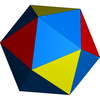(3.3.3.3.3)(332)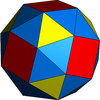(3.3.3.3.4)(432)(3.3.3.3.5)(532)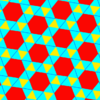3.3.3.3.6(632)3.3.3.3.7(732)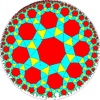3.3.3.3.8(832)

• ccw and cw spinning snub dodecahedron

Wikimedia Foundation. 2010.

### Look at other dictionaries:

• Snub cube — (Click here for rotating model) Type Archimedean solid Uniform polyhedron Elements F = 38, E = 60, V = 24 (χ = 2) Faces by sides (8+24){3}+6{4} …   Wikipedia

• Dodecahedron — Regular Dodecahedron (Click here for rotating model) Type Platonic solid Elements F = 12, E = 30 V = 20 (χ = 2) Faces by sides 12{5} …   Wikipedia

• Snub dodecadodecahedron — Type Uniform star polyhedron Elements F = 84, E = 150 V = 60 (χ = −6) Faces by sides 60{3}+12{5}+12{5/2} Wythoff symbol …   Wikipedia

• Snub polyhedron — A snub polyhedron is a polyhedron obtained by adding extra triangles around each vertex. Chiral snub polyhedra do not have reflection symmetry and hence have two enantiomorphous forms which are reflections of each other. Their symmetry groups are …   Wikipedia

• Snub disphenoid — Infobox Polyhedron with net Polyhedron Type=Johnson J83 J84 J85 Face List=4+8 triangles Edge Count=18 Vertex Count=8 Symmetry Group= D2d | Vertex List=4(34) 4(35) Dual= Property List=convex, deltahedron Net In geometry, the snub disphenoid is one …   Wikipedia

• Disdyakis dodecahedron — Click on picture for large version Spinning version Type Catalan Face polygon scalene triangle Faces 48 Edges …   Wikipedia

• Compound of two snub dodecahedra — Type Uniform compound Index UC69 Polyhedra 2 snub dodecahedra Faces 40+120 triangl …   Wikipedia

• Truncated great dodecahedron — Type Uniform star polyhedron Elements F = 24, E = 90 V = 60 (χ = −6) Faces by sides 12{5/2}+12{10} Wythof …   Wikipedia

• Liste Des Polyèdres Uniformes — Les polyèdres uniformes et les pavages forment un groupe bien étudié. Ils sont listés ici pour une comparaison rapide de leurs propriétés et de leurs noms de schéma variés ainsi que de leurs symboles. Cette liste inclut : tous les 75… …   Wikipédia en Français

• Liste des polyedres uniformes — Liste des polyèdres uniformes Les polyèdres uniformes et les pavages forment un groupe bien étudié. Ils sont listés ici pour une comparaison rapide de leurs propriétés et de leurs noms de schéma variés ainsi que de leurs symboles. Cette liste… …   Wikipédia en Français